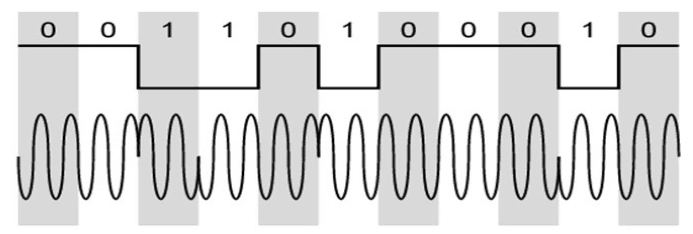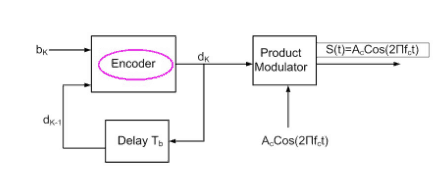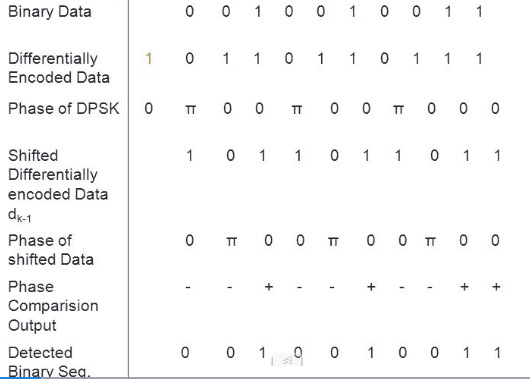# Differential Phase Shift Keying(DPSK)

## Differential Phase Shift Keying

### Definition :

Differential phase shift keying (DPSK) is a common type of phase modulation that conveys data by changing the phase of the carrier wave.

In DPSK the phase of the modulated signal is shifted relative to the previous signal element.The signal phase follows the high or low state of the previous element.

DPSK does not need a synchronous (coherent) carrier at the demodulator.

The input sequence of binary bits are modified such that the next bit depends upon the previous bit.

Therefore, in the receiver, the previous received bits are used to detect the present bit.

The following figure.1 shows the waveform of DPSK.Fig.1 : DPSK Waveform

It cab seen from the above figure that, when the data bit is Low i.e., ‘0’,  the phase of the signal is not reversed, and continued as it was.

When the data is a High i.e., ‘1’, the phase of the signal is reversed.

If we observe the above waveform, we can say that the High state represents an M in the modulating signal and the Low state represents a W in the modulating signal.

### DPSK Modulator

DPSK is a technique of BPSK, in which there is no reference phase signal.

In this case, the transmitted signal itself used as a reference signal.

The following  Fig.2 shows a DPSK Modulator.Fig.2 : DPSK Modulator

The serial data input b(t) is applied to the input of the encoder.

The output of the encoder is applied to one of the input of the product modulator.

To the other input of this product modulator , a sinusoidal carrier of fixed amplitude and frequency is applied.

The relationship between the binary sequence and its deferentially encoded version is shown in table.1 for a assumed data sequence 0 0 1 0 0 1 0 0 1 1 1.Table.1 : Differentially encoded sequences with phase

In this illustration it has been assumed that the encoding has been done in such a way that transition in the given binary sequence with respect to the previous encoded bit is represented by a symbol ‘0’ and no transition by symbol ‘1’.

It may be noted that an extra bit( symbol 1) has been arbitrarily added as an initial bit. This is essential to determine the encoded sequence.

The phase of the generated DPSK signal has been shown in the third row of table.1.

### DPSK Demodulator

In DPSK demodulator, the phase of the reversed bit is compared with the phase of the previous bit.

For detection of the differentially encoded PSK (DPSK) , we can use the receiver arrangement as shown in Fig.3 below.Fig.3 : DPSK Demodulator

The received DPSK signal is applied to one input of the multiplier and a delayed version of the received DPSK  signal by the time interval Tis applied to the other input of the multiplier.

The delayed version of the received DPSK signal ( in the absence of noise) has been shown in the 4th row of table.1.

The output of the difference is proportional to cos(Φ) , here Φ is the difference between the carrier phase angle of the received DPSK signal and its delayed version, measured in the same bit interval.

The phase angle of the DPSK signal and its delayed version have been shown in 3rd and 5th rows respectively.

The phase difference between the two sequences for each bit interval is used to determine the sign of the phase comparator output.

When Φ =0, the integrator output is positive whereas when Φ=π, the integrator output is negative.

By comparing the integrator output with a decision level of zero volt, the decision device can reconstruct the binary sequence by assigning a symbol ‘0’ for negative output and a symbol ‘1’ for positive output.

The reconstructed binary data is shown in the last row of the table.1.

It is thus seen that in the absence of noise, the receiver can reconstruct the transmitted data exactly.